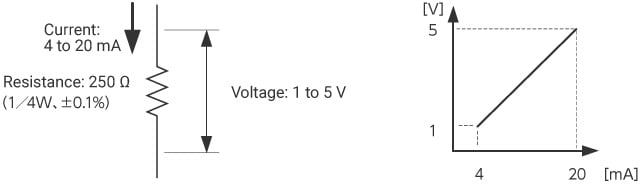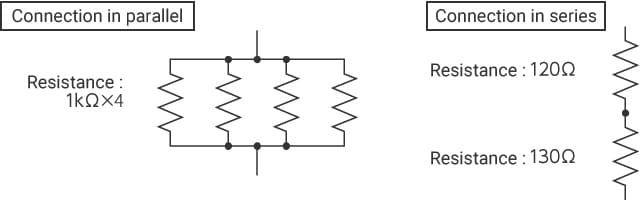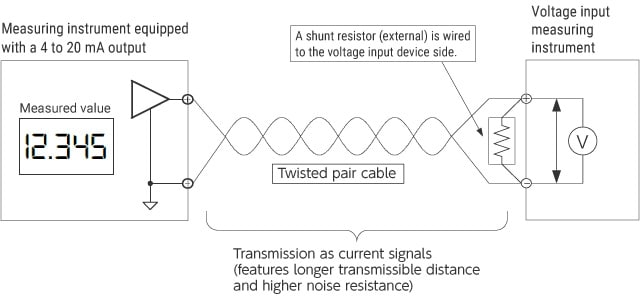# How to Select a Shunt Resistor

A shunt resistor is a resistor designed to detect current in a circuit. This resistor is also simply called a “shunt” and its applications include conductivity testing such as various evaluation tests for batteries. For example, in an overcharging or overdischarging test for batteries, a shunt resistor is inserted into the circuit to measure the voltage across the shunt resistor in order to measure the flowing current. This section explains a key point in selecting a shunt resistor and where to wire it.

## Selection of resistance and rating

Generally, a resistance of 250 Ω is used to convert 4 to 20 mA to voltage because it converts current to 1 to 5 V, which is easy to handle. Since the power consumption is (5 V × 20 mA) = 0.1 W at maximum, a rating of 1/4 W or more is used. As the tolerance, ±0.1% is selected in general, although it depends on the required conversion precision.## A resistance of 250 Ω

250 Ω is not a standard resistance value and thus, is not available as a general-purpose resistance; however, it can be created by combining resistances. For example, 250 Ω can be created by connecting four 1 kΩ resistances in parallel or connecting a 120 Ω resistance and a 130 Ω resistance in series.Q & A

• Q:Is it always necessary to use a resistance of 250 Ω?
• A:As long as the resistance is equal to or less than the maximum load resistance of the output device, it does not have to be 250 Ω. For example, if you use a 100 Ω resistance, a current output of 4 to 20 mA is converted to a voltage of 0.4 to 2 V, which can then be converted to an actual measured value through scaling.

## Where to wire a shunt resistor

A shunt resistor is wired to the voltage input device side in order to transmit output as current signals between devices. Transmission as current signals (4 to 20 mA) enables you to make more use of its advantages (such as longer transmissible distance and higher noise resistance). On the contrary, wiring a shunt resistor to the 4 to 20 mA output device side results in voltage transmission. For wiring between devices, twisted pair cables are used.INDEX